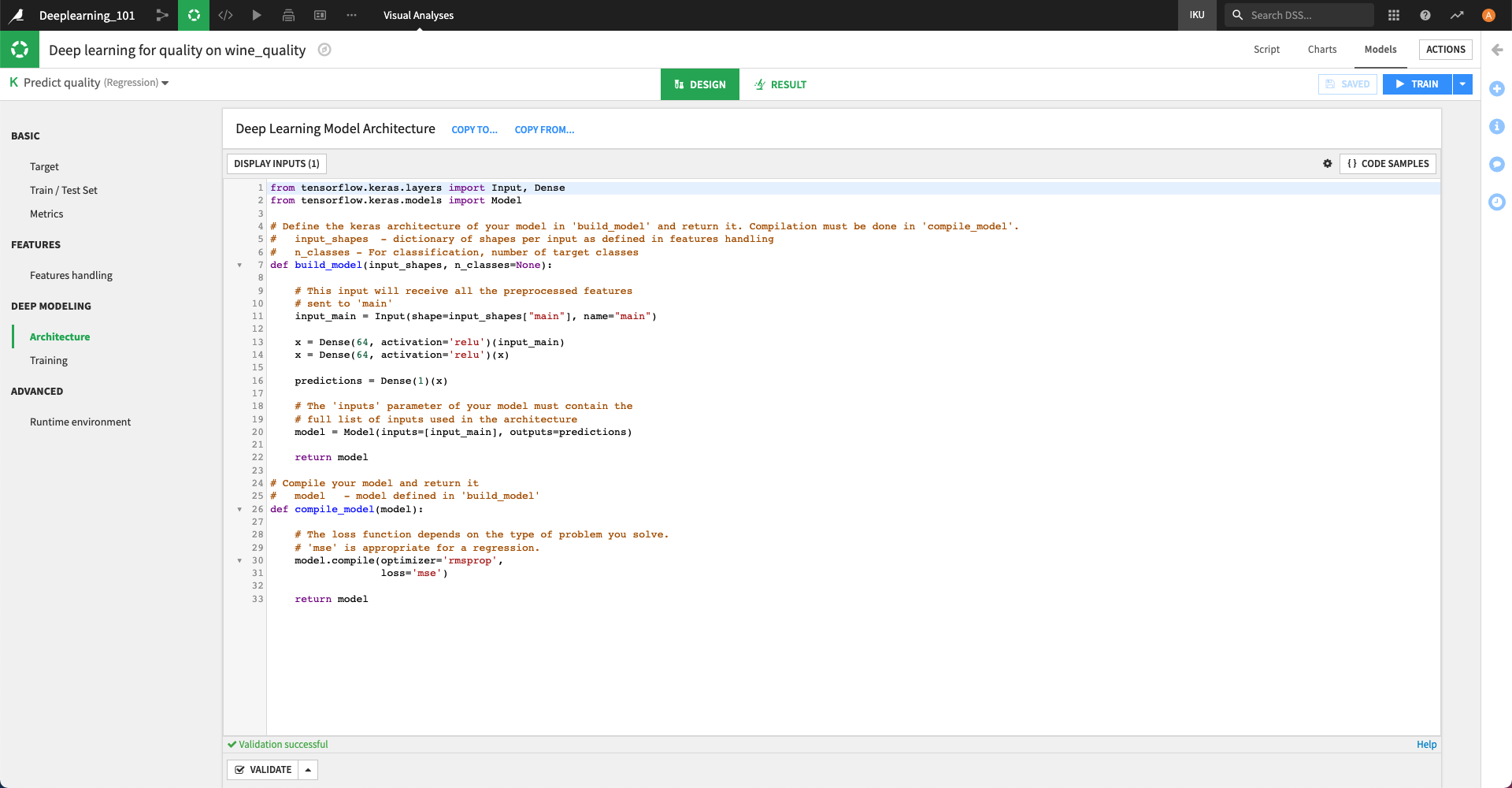# Model architecture¶

When creating a Deep Learning model, you need to write the Architecture of the Neural Network. To do so, you must fill two Python functions defined in the “Architecture” tab of the settings.## Build Keras model¶

The build_model function needs to return an instance of the Model class.

This function takes two parameters:

• input_shapes - a dictionary of the shapes of the input tensors

• n_classes - the number of classes to predict (for classification models only)

### input_shapes¶

Advanced models can have multiple inputs (see Multiple inputs for more information). Each input has a name.

The input_shapes variable is a dictionary indexed by input name. In most cases, the shape of the inputs is unknown prior to preprocessing, which will create a variable number of columns (e.g. dummification, vectorization …). Thus, they are provided to you to assist when building your model. If you haven’t used multi-input features, you only have a main input. Thus, to know the shape of your input tensor, simply use input_shapes["main"]

For example,

input_main = Input(shape=input_shapes["main"])
x = Dense(64, activation="relu")(input_main)
...


### n_classes¶

n_classes - the number of target classes when performing multiclass classification

### Layer dimensions¶

You need to be careful with the dimensions of the last layer of your network:

• For regression, the last layer needs to have a dimension equal to 1, and it should generally not have any activation.

• For binary classification, the last layer should be either: dimension equal to 1 and sigmoid activation, or dimension equal to 2 and softmax activation.

• For multiclass classification, the last layer should have a dimension equal to the number of target classes, and a softmax activation.

If this is not respected, training will either fail (mismatch in dimension) or give inconsistent results (when using an incorrect activation the result may not be a probability distribution).

## Compile the model¶

The compile_model function takes the previously created model as input and calls its compile method. It is separated from the build_model function, as DSS may need to manipulate the model between creation and compilation, in particular for multi-GPU training.

The compile function also optionally accepts a list of metrics to track during training. DSS also always computes some metrics (see Advanced topics for the list) that will be available in TensorBoard afterwards.

The two arguments that need to be carefully provided are:

• optimizer, which is the method used to optimize the model

• loss function, which is the function optimized during training. Loss functions are specific to the prediction type (Regression/Classification). By default DSS selects a suitable one based on the prediction type guessed in the analysis.